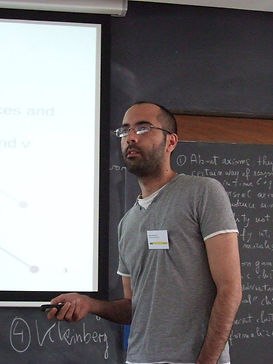Mr. Einstein once said "Imagination is more important than knowledge". I have nothing to add.

Welcome to my homepage!

## MY LATEST RESEARCHIrregularity Matrix in algebraic graph theory is my most recent toy! This simple graph-derived matrix is defined based on the difference between node degrees i.e. the element (i,j) of the matrix is the absolute difference between degrees of vertices i and j. In other words, it numerize the irregularity of a graph and can help studying Highly Irregular Graphs.Embedding problems (specially graph embedding) have always been attractive to me. One of my current research interests is the problem of Geometric Representation of graphs in which any vertex of a graph is mapped to a unique coordination in k-dimensional Euclidean space such that the topology of the graph is preserved.

Well ... at least fits the background image!